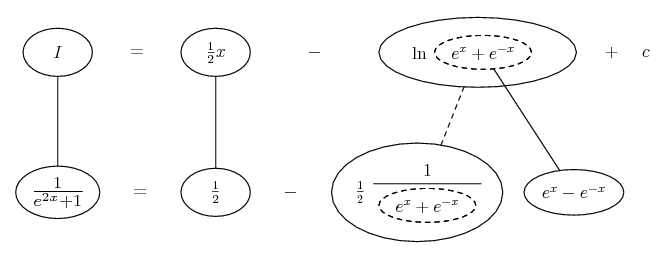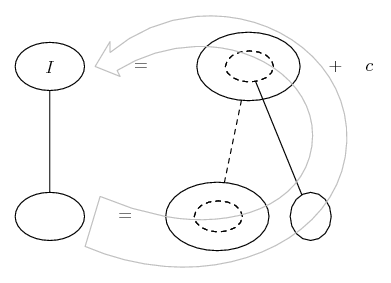# Thread: integration involving exponentials

1. ## integration involving exponentials

Hi, I have a question and the answer but i am slightly uncertain how the answer is achieved and was wondering if someone could please explain it to me.

Q: Using the fact that 2/(e^[2x]+1)=1 - (e^[2x]-1)/(e^[2x]+1)

integrate 1/(e^[2x]+1) with respect to x

A: 1/2{x-ln(e^x+e^-x)}+c

any help would be great thanks

2.Originally Posted by wahhdoeHi, I have a question and the answer but i am slightly uncertain how the answer is achieved and was wondering if someone could please explain it to me.

Q: Using the fact that 2/(e^[2x]+1)=1 - (e^[2x]-1)/(e^[2x]+1)

integrate 1/(e^[2x]+1) with respect to x

A: 1/2{x-ln(e^x+e^-x)}+c

any help would be great thanks
I don't think that any particularly clever idea is required to solve this integral, just substitute $\displaystyle z := e^{2x}+1$, thus $\displaystyle dz=2e^{2x}\,dx$ and therefore $\displaystyle dx=\frac{dz}{2(z-1)}$.
This gives

$\displaystyle \int \frac{1}{e^{2x}+1}\, dx=\frac{1}{2}\int \frac{dz}{z(z-1)}=\frac{1}{2}\int\left[\frac{1}{z-1}-\frac{1}{z}\right]\,dz=\ldots$

3. Although perhaps they meant...

$\displaystyle \frac{1}{e^{2x} + 1}$

$\displaystyle =\ \frac{1}{2}\ [1\ -\ \frac{e^{2x} - 1}{e^{2x} + 1}]$

$\displaystyle =\ \frac{1}{2}\ [1\ -\ \frac{e^x - e^{-x}}{e^{x} + e^{-x}}]$

And now the top is the derivative of the bottom. So you can do...... where... is the chain rule. Straight continuous lines differentiate downwards (integrate up) with respect to x, and the straight dashed line similarly but with respect to the dashed balloon expression (the inner function of the composite which is subject to the chain rule).

The general drift being..._________________________________________

Don't integrate - balloontegrate!

Balloon Calculus; standard integrals, derivatives and methods

Balloon Calculus Drawing with LaTeX and Asymptote!

#### Search Tags

exponentials, integration, involving